×

Search anything:

# PageRank

#### Machine Learning (ML) Algorithms Natural Language Processing (NLP)FREE BOOK -> Problems for the day before your Coding Interview (on Amazon)

Reading time: 35 minutes | Coding time: 10 minutes

PageRank algorithm is most famous for its application to rank Web pages used for Google Search Engine. It was developed by one the founders of google Larry Page and was named after the same. It is an algorithm to assign weights to nodes on a graph based on the graph structure.

# Introduction

PageRank algorithm follows a graph based approach in which the edges are weighted in order to prioritize the important webpages acoording to the query searched.
The basic concept implies that if a Node(Webpage) is important than the links or the nodes connected to that particular node also becomes important. PageRank is calculated from a webGraph containing all the Webpages as nodes and hyperlinks as edges. A node has two types of hyperlinks knwon as:

Incoming hyperlink refers to all the links that are directed towards the node from other nodes while Outgoing hyperlinks are those links that are directed from that particular node towards the other nodes.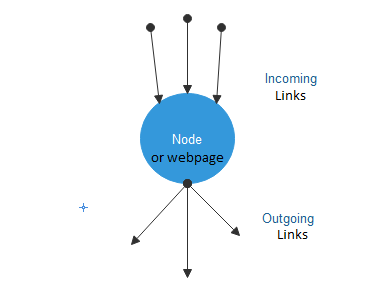## Weighing structure

There are few important points to consider while learning PageRank :
1.The links are weighted from 0-10. Where 0 is assigned to the spam pages and 10 is assigned to the most relevant pages.
2.Importance of a WebPage is calculated by considering the links pointing towards it. More the links point towards it, more the importance increases and vice cersa.
3.PageRank is query independent process thus it is much more faster than other dependent methods. Query independent means that the popularity score is calculated offline so that at runtime no time is spent in computation of the popularity scores for webpages.

## Algorithm

A PageRank algorithm takes into consideration the incoming links, outgoing links and the backlinks in order to calculate the rank of a page.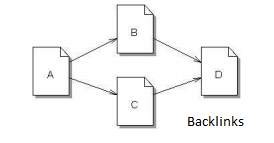A Backlink is just another edge that connects the current node from the previous node.

PageRank considers each node or link as a vote and works on the assumption that some of these votes are important to the others. Unlike other algorithms, it considers both uplink and downlink. It ranks each page indivisualy.where, d(damping factor) = [0,1]
It is also the source method for other summarization methods: TextRank and LexRank.

## Computation

Let us assume that there are four web Pages namely A,B,Cand D. Multiple outbound links from one page to another page are treated as a single link. as d lies in [0,1]. Thus each page will have initial value as 0.25.

1.PR(A)=PR(B)+PR(B)+PR(C), Assuming that A has link to B,C and D ,are the only links.

If the pages are interlinked to other pages:where L(A),L(B) and L(C) is the count of outbound or outgoing links from node A, node B and node C respectively.

1. Iterate over the set of pages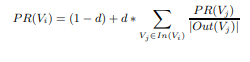where d is the damping factor.

Let us assume another example where B has a link to A and C, D has a link to the rest three pages and page C has a link to page A.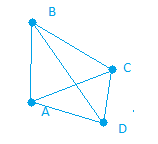After the first iteration we will have the following graph, Where B tranfers its value to its links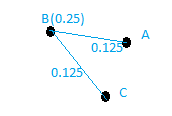Similarly, C and A link will weigh 0.25 as C transfers its value to A and D has 0.083 weigh on all the links from it by transfering its 0.25 to all the three nodes it is connected to.Finally, A will have 0.458 weigh after the first iteration.

``````PR(A)=PR(B)/L(B)+PR(C)/L(C)+PR(D)/L(D)
PR(A)=0.25/2 + 0.25/1 + 0.25/3
PR(A)=0.458
``````

### Applications

The applications of PageRank algorithm are:

3. Subroutine in Text summarization algorithms like TextRank

## Pseudocode

``````import numpy as np
from scipy.sparse import csc_matrix

def pageRank(G, s = .85, maxerr = .0001):
"""
Computes the pagerank for each of the n states
Parameters
----------
G: matrix representing state transitions
Gij is a binary value representing a transition from state i to j.
s: probability of following a transition. 1-s probability of teleporting
to another state.
maxerr: if the sum of pageranks between iterations is bellow this we will
have converged.
"""
n = G.shape

# transform G into markov matrix A
A = csc_matrix(G,dtype=np.float)
rsums = np.array(A.sum(1))[:,0]
ri, ci = A.nonzero()
A.data /= rsums[ri]

# bool array of sink states
sink = rsums==0

# Compute pagerank r until we converge
ro, r = np.zeros(n), np.ones(n)
while np.sum(np.abs(r-ro)) > maxerr:
ro = r.copy()
# calculate each pagerank at a time
for i in xrange(0,n):
Ai = np.array(A[:,i].todense())[:,0]
# account for sink states
Di = sink / float(n)
# account for teleportation to state i
Ei = np.ones(n) / float(n)

r[i] = ro.dot( Ai*s + Di*s + Ei*(1-s) )

# return normalized pagerank
return r/float(sum(r))

if __name__=='__main__':
# Example extracted from 'Introduction to Information Retrieval'
G = np.array([[0,0,1,0,0,0,0],
[0,1,1,0,0,0,0],
[1,0,1,1,0,0,0],
[0,0,0,1,1,0,0],
[0,0,0,0,0,0,1],
[0,0,0,0,0,1,1],
[0,0,0,1,1,0,1]])
print pageRank(G,s=.86)
``````

## Quick Implementation using Neworkx

Now we will try to make our own WWW using networkx

``````import networkx as nx
import matplotlib.pyplot as plt
import numpy as np
DG = nx.DiGraph()
nx.draw(DG,with_labels=True)
plt.show()
``````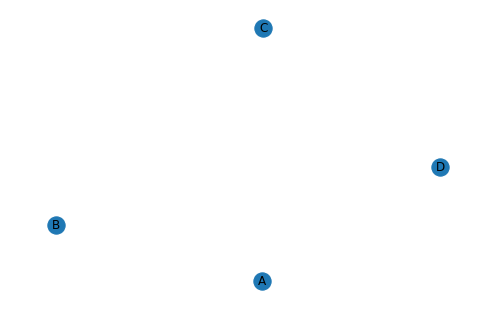As there are no links between the nodes initially, thus they will be equally important

``````pr=nx.pagerank(DG, alpha=0.85)
pr
``````

Output (pr):

``````{'A': 0.25, 'B': 0.25, 'C': 0.25, 'D': 0.25}
``````

Now we initialize the links in our graph

``````DG.add_weighted_edges_from([("A", "B", 1), ("B", "C", 1),("C","D",1),("D","A",1)])
nx.draw(DG,with_labels=True)
plt.show()
``````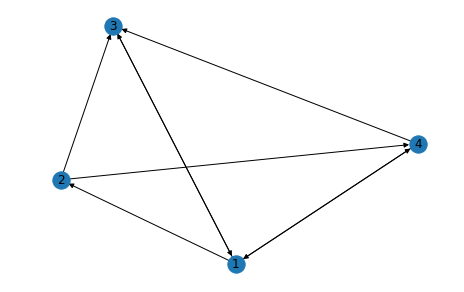``````B=np.matrix([(0,0,1,0.5),(1/4,0,0,0),(1/6,0.5,0,0.5),(1/3,0.5,0,0)])
B
``````

Output

``````        [0.25      , 0.        , 0.        , 0.        ],
[0.16666667, 0.5       , 0.        , 0.5       ],
[0.33333333, 0.5       , 0.        , 0.        ]])
``````
``````#Applying for damping factor(alpha)=1(
pr=nx.pagerank(DG_test,alpha=1)
pr
``````

Output (pr)

``````{1: 0.38709615908859496,
2: 0.12903204605249047,
3: 0.29032302109901886,
4: 0.193548773759895}
``````
``````#Rank vector for k=1000 iterations
np.array((B**1000)*A.T)
``````

Output

``````array([[4.82572159e-48, 4.09722135e-48, 5.23156516e-48, 4.56508003e-48],
[1.34419179e-48, 1.14127001e-48, 1.45723843e-48, 1.27159079e-48],
[3.06060181e-48, 2.59856746e-48, 3.31799867e-48, 2.89529595e-48],
[2.54109775e-48, 2.15748874e-48, 2.75480428e-48, 2.40385078e-48]])
``````
``````G=nx.fast_gnp_random_graph(10,0.5,directed=True)
nx.draw(G,with_labels=True)
plt.show()
``````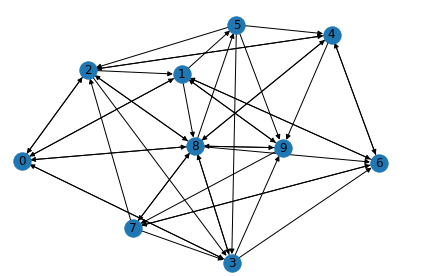Now predicting the most important node

``````pr=nx.pagerank(G,alpha=0.85)
rank_vector=np.array([[*pr.values()]])
ImpNode=np.argmax(rank_vector)
print("The most important website is {}".format(ImpNode))
``````

The most important website is 8#### Ashutosh Vashisht

B.Tech (IT) Student at Amity School of Engineering and Technology